## Five Disks Problem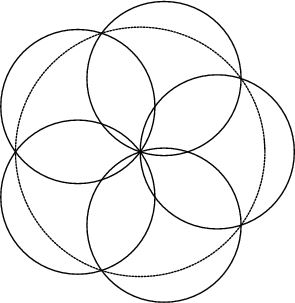Given five equal Disks placed symmetrically about a given center, what is the smallest Radiusfor which the Radius of the circular Area covered by the five disks is 1? The answer is, where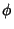is the Golden Ratio, and the centers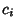of the disks, ..., 5 are located at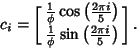The Golden Ratio enters here through its connection with the regular Pentagon. If the requirement that the disks be symmetrically placed is dropped (the general Disk Covering Problem), then the Radius for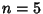disks can be reduced slightly to 0.609383... (Neville 1915).

See also Arc, Disk Covering Problem, Flower of Life, Seed of Life

References

Ball, W. W. R. and Coxeter, H. S. M. The Five-Disc Problem.'' In Mathematical Recreations and Essays, 13th ed. New York: Dover, pp. 97-99, 1987.

Neville, E. H. On the Solution of Numerical Functional Equations, Illustrated by an Account of a Popular Puzzle and of its Solution.'' Proc. London Math. Soc. 14, 308-326, 1915.